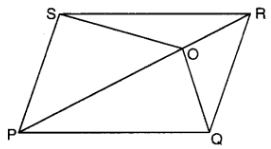Maths / Areas Of Parallelograms And Triangles / Median and Altitude of Triangle

QUESTION

In the given figure, O is any point on diagonal PR of parallelogram PQRS. Prove thatEXPLANATION
Explain TypeExplanation Content
Text

Given : PQRS is a parallelogram.

Proof : SQ is a diagonal.

M is the midoint of SQ.

OM is a median of

Image required

(Median divides the triangle in 2 equal areas)  .............. (1)

Also,

In  is median.

Subtracting (1) and (2), we get

Now, PR is a diagonal.

(Diagonal divides the parallelogram in 2 equal areas)

Hence proved

TestimonialsPARENT FEEDBACK c/o Yogansh Nyasi C/o ABHYAS Academy
7th
Abhyas is an institute of high repute. Yogansh has taken admission last year. It creates abilities in child to prepare for competitive exams. Students are motivated by living prizes on basis of performance in Abhyas exams. He is satisfied with institute.